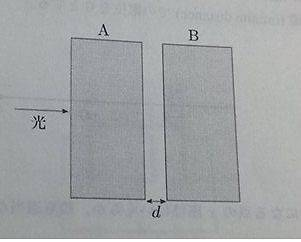# Light interference

## Homework Statement[/B]
Find condition of d that constructive interference occurs
with m=1,2,3,...
a)##(m-\frac{1}{2})\frac{\lambda}{2}##
b)##m\frac{\lambda}{2}##
c)##(m-\frac{1}{2})\lambda##
d)##m\lambda##

## Homework Equations

optical path difference (OPD) must equal ##m\lambda##

## The Attempt at a Solution

The optical path difference i finded is:
$$2d+\frac{\lambda}{2}=m\lambda$$
So ##d=(m-\frac{1}{2})\frac{\lambda}{2}## and I choose A
But the answer is B)## d=m\lambda##
Where is wrong?
Thanks for helping

•